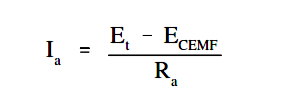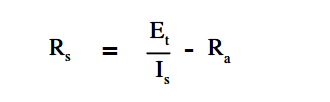# Starting of DC Motors

At the moment a DC motor is started the armature is stationary and there is no counter EMF being generated. The only component to limit starting current is the armature resistance, which, in most DC motors is a very low value (approximately one ohm or less), as shown in below Equation.In order to reduce this very high starting current, an external resistance must be placed in series with the armature during the starting period. To show why this is essential, let us consider a 10-hp motor with an armature resistance of 0.4 ohms. If the motor were supplied by a 260 VDC source, the resulting current would be as shown in Equation.Ia =  ( 260 – 0 ) / 0.4  =  650 amps

This large current is approximately twelve times greater than actual full-load current for this motor. This high current would, in all probability, cause severe damage to the brushes, commutator, or windings. Starting resistors are usually incorporated into the motor design to limit starting current to 125 to 200 percent of full load current.

The amount of starting resistance necessary to limit starting current to a more desirable value is calculated using Equation.where

Rs = starting resistance
Et = terminal voltage
Is = desired armature starting current
Ra = armature resistance

Example:

If the full load current of the motor mentioned previously is 50 amps, and it is desired to limit starting current to 125% of this value, find the required resistance that must be added in series with the armature.Rs =  { 260 / (125 x 50) } – 0.4

Rs = 3.76 Ω

The starting resistors are used in a DC motor by placing them in the starting circuit of the motor controller that is used to start the DC motor. Starting resistors are normally of variable resistances, with the value of resistance in the circuit at any time being either manually or automatically controlled.

The maximum amount of resistance will always be inserted when the motor is first started. As the speed of the motor increases, counter EMF will begin to increase, decreasing armature current. The starting resistors may then be cut out, in successive steps, until the motor reaches full running speed.

DC Motor Ratings

The nameplate ratings of a DC motor refer to the conditions of voltage, current, speed, and power at which the motor is normally operated. The principal rating is known as the continuous rating, which is the rating described on the nameplate of a motor. The continuous power rating is a thermal rating. At this power, the motor can be operated for long periods of time without a large rise in temperature and beyond the limits of the conductor insulating material, bearings and other components, which are greatly affected by temperature.

The speed rating of a DC motor is often given on the nameplate. This speed is the upper limit at which a motor can be operated without mechanical damage occurring.

Don't Miss Our Updates
Be the first to get exclusive content straight to your email.
We promise not to spam you. You can unsubscribe at any time.